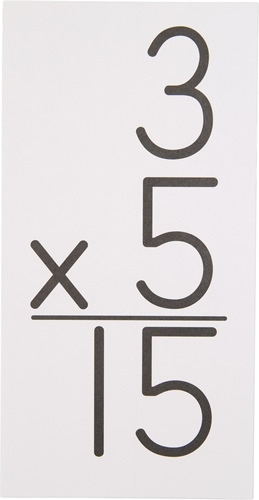• # Math

Eureka Math is the district curriculum used to teach the Common Core Math Standards. Eureka is designed to make real life connections to the math students learn. It focuses on problem solving, and prepares students to ask and answer, "Why?" They will not be simply memorizing math facts, but instead, they will be expected to explain their reasoning in a collaborative classroom setting. Through this, it is intended that students will have advanced mental math skills.   Third graders will focus on the following module (chapter) topics:

• Properties of Multiplication and Division and Solving Problems with Units of 2–5 and 10
• Place Value and Problem Solving with Units of Measure
• Multiplication and Division with Units of 0, 1, 6–9, and Multiples of 10
• Multiplication and Area
• Fractions as Numbers on the Number Line
• Collecting and Displaying Data
• Geometry and Measurement Word Problems